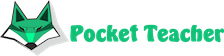# How algebra differs from arithmetic

Both sciences are different sides of the same coin. Arithmetic has a thorough knowledge of numbers, it gives an ability to use it for any calculations in everyday life. The basics of arithmetic is given in early childhood. Parents teach kids to count. The child masters elementary arithmetic at school and can calculate algebra problems of different complaxity. Algebra studies the objective properties of idealized objects using numbers and letters. This is the second mathematical step in terms of complexity.

## Difference

Arithmetic translates from Greek as "number", which fully reveals its essence. It studies numbers, analyzes actions with them. Higher arithmetic that uses real, irrational numbers is known as number theory.

Algebra is an Arabic term borrowed from medicine. It translates as "the connection of broken parts." This science deals not only with numbers, but with a wide variety of sets (not necessarily numeric, but also alphabetic). It solves equations, systems of equations, studies symmetry, constants, logical operations (Boolean algebra).

In other words, algebra is the sister of arithmetic, dealing with more complex objects. They have common rules for solving problems. Today you can find math solver on our site. It is popular among schoolchildren and their parents, students and applicants.

Example:

1+3 =3+1. This is purely arithmetic numerical equality, showing some regularity.

а+b = b+а. It is an algebraic equation that works for a variety of situations based on certain patterns. Algebra is a set of conditions that are valid for any numbers.

## Main comparative characteristics

The main differences between related sciences are as follows:

1. Arithmetic is the most important part of mathematics, appealing with numbers, adding, multiplying, subtracting and dividing them. Algebra is another mathematical branch that solves the set of problems using not only numbers, but also letters (unknown quantities). It relies on the general rules of calculations.

2. Arithmetic is the first stage, mathematics of primary school. Algebra is the second one. It is related to secondary school education.

3. Arithmetic uses operations with known numbers as a technique. Algebra is actions with abstract values that have a common meaning.

4. Arithmetic uses four basic mathematical operations as a solution tool: addition, subtraction, multiplication, division. Algebra is operations with numbers and letters (sets, variables) based on the general rules of mathematics.

5. The way to solve arithmetic is to search for an answer according to the conditions of a problem. The result - small numbers. Algebra is the use of standard algorithms for elementary algebra (algebraic formulas).

Arithmetic and algebra are two stages of the most exact science of mathematics, acting in the same direction.Our artificial intelligence solves complex math problems in seconds.

We will solve your exam, homework, olympiad problems with detailed steps. You will need just to copy the solution.